Updating search results...

# 93 Results

View
Selected filters:
• problem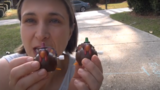Unrestricted Use
CC BY
Rating
0.0 stars

This lesson is Day 8 in a series of 12 lessons around fraction equivalences and comparisons.

This video lesson introduces the number as a tool for representing and comparing fractions. Students will need a black piece of a paper and a pencil with a good eraser.

Subject:
Mathematics
Material Type:
Activity/Lab
Interactive
Lesson
Simulation
Author:
Dawne Coker
06/25/2020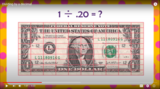Unrestricted Use
CC BY
Rating
0.0 stars

This video shows HOW and WHY we can shift decimals to make whole numbers when dividing.

Subject:
Mathematics
Material Type:
Activity/Lab
Diagram/Illustration
Lesson
Teaching/Learning Strategy
Author:
Dawne Coker
06/26/2020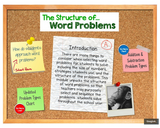Only Sharing Permitted
CC BY-ND
Rating
0.0 stars

This Thinglink provides a series of brief videos addressing a) common areas of difficulty related to problem solving and b) an overview of additional and subtraction problem types.

Subject:
Mathematics
Material Type:
Activity/Lab
Diagram/Illustration
Interactive
Reference Material
Simulation
Author:
Dawne Coker
07/01/2020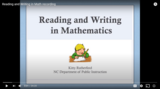Only Sharing Permitted
CC BY-NC-ND
Rating
0.0 stars

This video has been created by NCDPI. It provides tips for integrating reading within the math block.

Subject:
Mathematics
Material Type:
Diagram/Illustration
Reference Material
Teaching/Learning Strategy
Author:
Kitty Rutherford
06/28/2020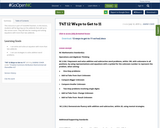Conditional Remix & Share Permitted
CC BY-NC-SA
Rating
0.0 stars

This resource is part of Tools4NCTeachers.

In this lesson, students will be figuring out the addends that add up to the correct sums. They will also be creating and solving equations with more than two addends.

Subject:
Mathematics
Material Type:
Assessment
Lesson
11/06/2019Unrestricted Use
CC BY
Rating
0.0 stars

This resource is from Tools4NCTeachers.In this lesson the students are introduced to measuring length with standard units as they attempt to create a bed for a queen. They will also measure the length of an object in both inches and centimeters.

Subject:
Mathematics
Material Type:
Activity/Lab
Lesson
Lesson Plan
Author:
DAWNE COKER
06/23/2020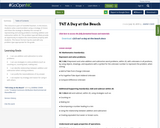Conditional Remix & Share Permitted
CC BY-NC-SA
Rating
0.0 stars

This resource is part of Tools4NCTeachers.

In this lesson, students solve a word problem using a strategy of choice and share the strategy to develop the concept of representing and solving problems involving addition and subtraction within 20. This problem type will likely provide an opportunity to explore the commutative property with students. This lesson format may be used with any problem type appropriate for the grade.

Subject:
Mathematics
Material Type:
Lesson
Lesson Plan
11/06/2019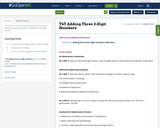Conditional Remix & Share Permitted
CC BY-NC-SA
Rating
0.0 stars

This resource is part of Tools4NCTeachers.

In this lesson, students explore approaches for adding three two-digit numbers.

Subject:
Mathematics
Material Type:
Lesson
Lesson Plan
11/06/2019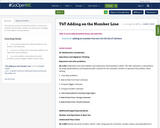Conditional Remix & Share Permitted
CC BY-NC-SA
Rating
0.0 stars

This resource is part of Tools4NCTeachers.

In this lesson students use the open number line and various strategies to solve addition story problems.

Subject:
Mathematics
Material Type:
Lesson
Lesson Plan
11/06/2019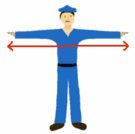Unrestricted Use
CC BY
Rating
0.0 stars

This lesson is from Tools4NCTeachers.In this lesson, students use a measuring tape to measure, record, and compare the lengths of their arm spans. Remix this lesson to include pictures of students in action!&nbsp;&nbsp;

Subject:
Mathematics
Material Type:
Activity/Lab
Lesson
Lesson Plan
Author:
DAWNE COKER
06/23/2020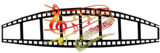Unrestricted Use
CC BY
Rating
0.0 stars

This resource is from Tools4NCTeachers.&nbsp;&nbsp;In this lesson, students determine whether or not they have enough money to purchase popcorn in addition to movie tickets. They then determine whether or not there will be change and if so, how much change they would receive. Printable student sheets are provided in this lesson.

Subject:
Mathematics
Material Type:
Activity/Lab
Lesson
Lesson Plan
Author:
DAWNE COKER
06/23/2020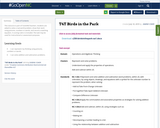Conditional Remix & Share Permitted
CC BY-NC-SA
Rating
0.0 stars

This resource is part of Tools4NCTeachers.

Students are asked to solve a real world problem, show their work using pictures, numbers or words, and record a matching equation. A scoring rubric is included. The task may be used for instructional or assessment purposes.

Subject:
Mathematics
Material Type:
Assessment
Formative Assessment
11/06/2019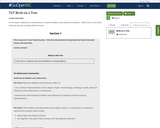Unrestricted Use
CC BY
Rating
0.0 stars

In this lesson, students use manipulatives to explore addition and subtraction situations.&nbsp; Math centers and center materials are also provided with this lesson.&nbsp;&nbsp;

Subject:
Mathematics
Material Type:
Activity/Lab
Lesson
Author:
DAWNE COKER
12/26/2019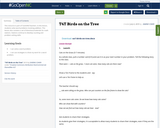Conditional Remix & Share Permitted
CC BY-NC-SA
Rating
0.0 stars

This resource is part of Tools4NCTeachers.

In this lesson, students solve word problems involving addition. This lesson also contains a set of directions/materials for math stations. Stations continue to develop counting and problem solving skills.

Subject:
Mathematics
Material Type:
Lesson
Lesson Plan
11/06/2019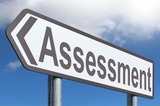Unrestricted Use
CC BY
Rating
0.0 stars

This resource is from Tools4NCTeachers.A Common Formative Assessment (CFA) provides a snapshot of current student understanding. It serves as a checkpoint, and may be used to guide future whole and small group instruction. This CFA addresses 2nd grade, Cluster 6&nbsp;standards.

Subject:
Mathematics
Material Type:
Assessment
Formative Assessment
Author:
DAWNE COKER
06/16/2020Unrestricted Use
CC BY
Rating
0.0 stars

This resource is from Tools4NCTeachers.A Common Formative Assessment (CFA) provides a snapshot of current student understanding. It serves as a checkpoint, and may be used to guide future whole and small group instruction. This CFA addresses 2nd grade, Cluster 8&nbsp;standards. &nbsp;Answer key is provided.

Subject:
Mathematics
Material Type:
Assessment
Formative Assessment
Author:
DAWNE COKER
06/16/2020Unrestricted Use
CC BY
Rating
0.0 stars

This resource is from Tools4NCTeachers.In this lesson, students will use manipulatives to solve subtraction problems within 10 that involve removing objects from a group. Remix this lesson to share your ideas for additional word problems, extensions, or student work-samples.

Subject:
Mathematics
Material Type:
Activity/Lab
Lesson
Lesson Plan
Author:
DAWNE COKER
06/08/2020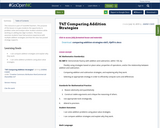Conditional Remix & Share Permitted
CC BY-NC-SA
Rating
0.0 stars

This resource is part of Tools4NCTeachers.

This purpose of this lesson is to give students the opportunity to problem solve and analyze other student solutions while working on adding two-digit numbers. This lesson assumes students have had previous experience with multiple addition strategies and that the class has posted strategy supports.

Subject:
Mathematics
Material Type:
Lesson
Lesson Plan
11/06/2019Unrestricted Use
CC BY
Rating
0.0 stars

This resource is from Tools4NCTeachers.&nbsp;&nbsp;In this lesson, students will create a survey question, collect data, create a bar graph, write and answer questions about the collected data.&nbsp; This lesson may take two days to complete.&nbsp; Printable, samples of student work, sample anchor charts, and extension ideas are included within this lesson.

Subject:
Mathematics
Material Type:
Activity/Lab
Lesson
Lesson Plan
Author:
DAWNE COKER
06/23/2020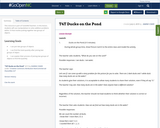Conditional Remix & Share Permitted
CC BY-NC-SA
Rating
0.0 stars

This resource is part of Tools4NCTeachers.

In this lesson, students will use manipulatives to solve addition problems within 10 that involve putting together two groups of objects.

Subject:
Mathematics
Material Type:
Lesson
Lesson Plan